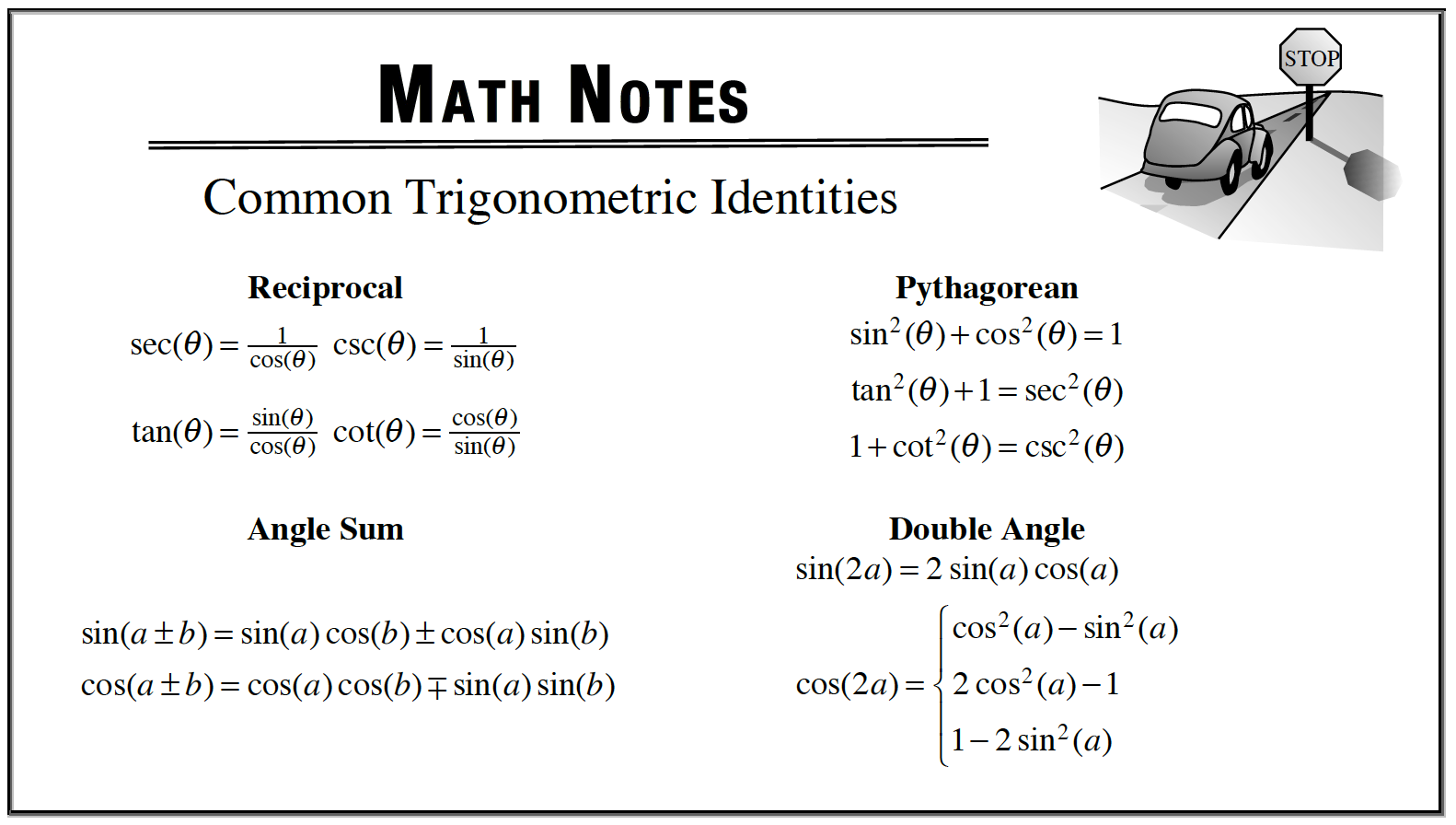### Home > APCALC > Chapter 6 > Lesson 6.5.1 > Problem6-176

6-176.

Evaluate the following derivatives. Use trigonometric substitutions to make your work easier. Homework Help ✎

1. $\frac { d } { d x } ( 4 \operatorname { cos } ( 2 x ) \operatorname { sin } ( 2 x ) )$

Use a trigonometric identity AFTER you find the derivative.$4\cos(2x)\sin(2x) =$
$2\sin(4x)$

2. $\frac { d } { d x } ( 6 \operatorname { cos } ^ { 2 } ( x ) + 6 \operatorname { sin } ^ { 2 } ( x ) )$

Simplify the expression using a trigonometric identity BEFORE you take the derivative.

3. $\frac { d } { d x } \operatorname { arccot } ( 2 ^ { 3 x } )$

Careful!

$\text{arccot}(x)\neq \frac{1}{\arctan(x)}$

$\text{arccot}(x)=\text{arctan}\left (\frac{1}{x}\right )$